# Average Cells Based On Multiple Criteria in Excel

• Last Updated : 23 Jun, 2021

Average a number expressing the central or typical value in a set of data, in particular the mode, median, or (most commonly) the mean, which is calculated by dividing the sum of the values in the set by their number. The basic formula for the average of n numbers x1,x2,……xn is

`A = (x1 + x2 ........xn)/ n`

In Excel, there is an average function for this purpose, but it can be calculated manually also using SUM and COUNT functions like this

Attention reader! Don’t stop learning now. If you are an Excel beginner (or an intermediate) and want to learn Excel, Geeksforgeeks brings the perfect course for you to start, Diving Into Excel

`= SUM(D1:D5)/COUNT(D1:D5) `

Here

1. SUM: This function is used to find the sum of values in the required range of cells.
2. COUNT: This function is used to get the count of the required range of cells containing numeric values.

### AVERAGE Function

Excel provides a direct function named AVERAGE to calculate the average (or mean) of the numbers in the range specified in the function’s argument. In this function, maximum of 255 arguments can be given (numbers / cell references/ ranges/ arrays or constants).

Syntax:

`= AVERAGE(Num1, [Num2], ...)`

Here

1. Num1 [Required]: Provide range, cell references, or the first number for calculating the average.
2. Num2, … [Optional]: Provide additional numbers or range of cell references for calculating the average.

Example:

`AVERAGE(C1:C5) `

This will give the average of numeric values in the range C1 to C5.

Note: In the above-mentioned methods, any criteria for calculating the average cannot be mentioned. For this excel provide another function called AVERAGEIF.

### Average Cells Based On One Criterion

Excel provides a direct function named AVERAGEIF to calculate the average (or mean) of the numbers in the range specified that meets particular criteria specified in the function’s argument.

Syntax:

`= AVERAGEIF(Range_Cells_For_Criteria, Criteria, [Range_Cells_For_Average])`

Here

1. Range_Cells_For_Criteria [Required]: Give the range of cells on which criteria are to be tested.
2. Criteria [Required]: This is the condition on which to decide the cells to average. The criteria can be given in the form of an expression(logical), text-value or number, or reference to a cell, e.g. 100 or “>100” or “Apple” or A7.
3. Range_Cells_For_Average [Optional]: Specify the cells on which the average needs to be calculated. Range_Cells_For_Criteria is used to find the average if it is not included.

Note:

1. An empty cell in average_range is ignored here.
2. Cells in the range that contain TRUE or FALSE are ignored here.
3. If the range is blank, return the #DIV0! Error value.
4. Empty cell in the criteria is treated as 0.

### Using Double Quotes (“”) In Criteria

In Excel, the text values are surrounded by double quotations (“”), but integers are not, in general. But when numbers are used in criteria(s) with a logical operator, the number and operator must also be wrapped in quotes.

Examples:

1. To calculate the average of only positive numbers excluding 0 in the range A2 to A6
= AVERAGEIF(A2:A6,”>0″) is correct.
= AVERAGEIF(A2:A6,>0) is incorrect as the logical operator should be included in double-quotes.

2. To get the average age (AGE is in column C) of the employees living in the city having id = 1 (CityId is in column D)
= AVERAGEIF(D2:D10, “=1”, C2:C10) is correct
= AVERAGEIF(D2:D10, 1, C2:C10) is also correct as here also D2 to D10 cells will be checked for value 1.

3. To get the average age (AGE is in column C) of the employees living in a city named Mumbai (City is in column B)
= AVERAGEIF(B2:B10, “Mumbai”, C2:C10) is correct
= AVERAGEIF(B2:B10, Mumbai, C2:C10) is incorrect as text should be included in double-quotes.

Example 1: To calculate the average of only positive numbers excluding 0 in the range A2 to A6.

Solution: In this example, a single criterion is given- “>0″. So all the numbers in the range that are greater than 0 come in the criteria. The average will be calculated as

`(12.7 + 87.2 + 100) / 3 = 66.66666667`

So, the average formula for the above example is

`AVERAGEIF(A2:A6,">0")`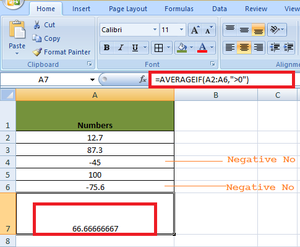Example 2: To get the average age (AGE is in column C) of the employees living in a city named Mumbai (City is in column B).

Solution: Let’s break the example into different parts so that the individual parts can then be substituted in the AVERAGEIF function

• Criteria: “Mumbai”.
• Cells for criteria: B2 to B6.
• Cells for average: C2 to C6 that meet the criteria.

The average age of the employees living in Mumbai

`= (65 + 45) /2 = 55`

So, the average formula for this example is

`AVERAGEIF(B2:B6,"Mumbai",C2:C6)`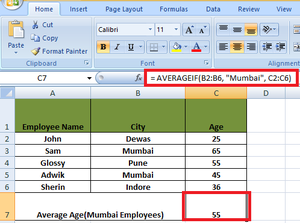### Average Cells Based On Multiple Criterion

Excel provides a direct function named AVERAGEIFS to calculate the average (or mean) of the numbers in the range specified that meets multiple criteria specified in the function’s argument.

Syntax:

```AVERAGEIFS(RangeForAverage,RangeForCriteria1,Criteria1,
[RangeForCriteria2, Criteria2],..)```

Here

1. RangeForAverage [Required]: Provide the cell range on which average needs to be computed.
2. RangeForCriteria1: Range on which criteria1 is applied.
3. Criteria1: The first criteria to apply on the RangeForCriteria1.

Note:

1. All ranges are required along with their criteria.
2. Criteria1 is required, the rest are optional.

Example 1: To calculate the average age of the employees living in Mumbai whose ages are > 50.

Solution: The following criteria are used to solve the problem

• RangeForAverage: C2 to C8.
• Criteria 1: “Mumbai”.
• RangeForCriteria1: B2 to B8.
• Criteria 2: “>50”.
• RangeForCriteria1: C2 to C8.

Here, the average is calculated on basis of two criteria- Employees living in Mumbai and employees whose Age > 50

`AVERAGEIFS(C2:C8,B2:B8,"Mumbai",C2:C8,">50")`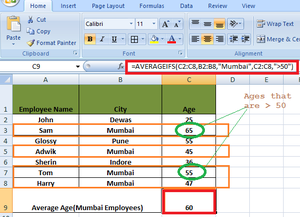Example 2: To get the average price of the product with Category ID 1 and having a Price greater than Rs 500/-.

Solution: The following criteria will be used for the AVERAGEIF function

• RangeForAverage: C2 to C11.
• Criteria 1: “=1”.
• RangeForCriteria1: B2 to B11.
• Criteria 2: “>500”.
• RangeForCriteria2: C2 to C11.

Here, the average is calculated on the basis of two criteria- Product having Category ID 1 and Price greater than 500

`AVERAGEIFSC2:C11,B2:B11,"=1",C2:C11,">500")`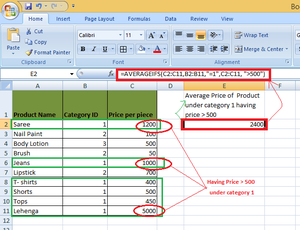### Using Values From Another Cell

The concatenation of text can be used to use the value from another cell. Concatenation is the process of connecting two or more values to form a text string. There are two ways for concatenation of the strings in Excel

1. Logical & operator can be used.
2. Concatenate function in Excel for concatenation of two texts.

Example: To get average marks (marks are in column B) of passed students having minimum passing marks value contained in cell D2.

Solution: In this example, the logical & operator will be used to concatenate the two conditions for finding the result

• Marks greater than 0.
• Minimum passing marks i.e. 10.

Here, D2 has a value of 10, so the minimum pass marks are 10, the following criteria will be used- “>=”&D2. This will concat “>=” & value contained in cell D2, i.e. 10. After concatenation, the criteria will become “>=10”.

So, in this example

• RangeForAverage- B2 to B12.
• Criteria1- Marks greater than 0.
• RangeForCriteria1- B2 to B12.

The following formula will be used to calculate the average

`=AVERAGEIFS(B2:B12,B2:B12,">=0"&D2)`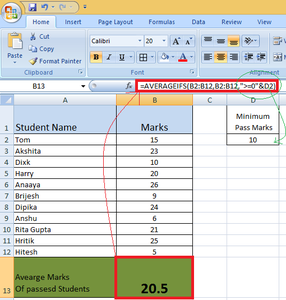### Wildcards

Excel provides wildcard characters that can be used in the criteria. Some wild cards are

1. * (asterisk): This symbol can be used to represent any number of characters.
2. ? (question mark): This symbol can be used to represent a single character.
3. ~ (tilde): This symbol is used before the above two wild cards and also tilde itself so that when there is a need to match a text containing * or? Or ~, Excel does not treat them as a wildcard. To treat them as normal characters in Excel criteria, the tilde is used before them.

Examples: There are the product names in column A (From A1 to A10) and their respective price in column C.

1. AVERAGEIFS(C2:C11, A:A11, “*a*”): To find the average price of all the products who have character ‘a’ anywhere in their name.
2. AVERAGEIFS(C2:C11, A:A11, “?a*”): To find the average price of all the products that have the second character ‘a’ in their name.
3. AVERAGEIFS(C2:C11, A:A11, “?aree”): To find the average price of all the products that have a single character before ‘aree’ in their name.
4. AVERAGEIFS(C2:C11, A:A11, ” ~*Saree “): To find the average price of all the products that have a name is *Saree.

Example: To find the average price of all the products that have character ‘a’ anywhere in their name.

Solution: In this example

1. Product Saree, Nail Paint, Jeans, and Lehenga contains the character ‘a’ in them.
2. The average prices for these products will be
`= (1200+ 100+ 1000+ 5000)/4 = 7300/4 = 1825 `

So, the complete formula for calculating the average is as follows

`AVERAGEIFS(C2:C11,A2:A11,"*a")`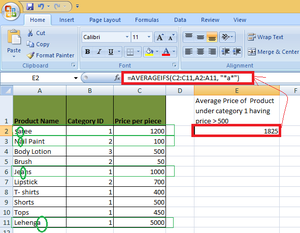### Conclusion

Using AVERAGE, it is possible to find average without any criteria. Using AVERAGEIF, the average can be calculated with particular criteria and using AVERAGEIFS, the average can be computed with multiple criteria(s).

My Personal Notes arrow_drop_up